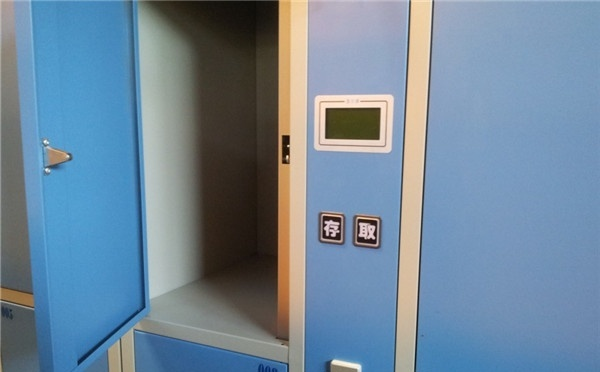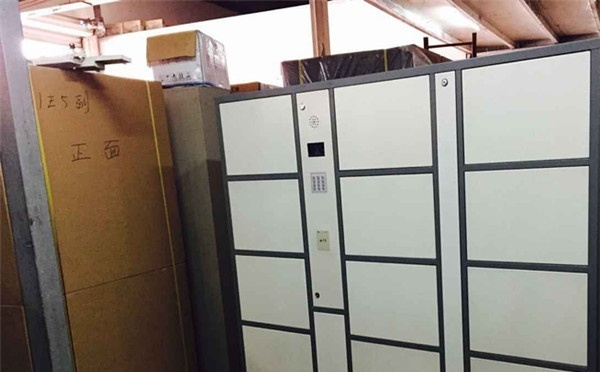|

# 什么是指纹储物柜 指纹储物柜厂家介绍

现在我们在很多的公共场所都能看到一种新型的储物柜—指纹储物柜，很多大型商城或者是家用都有很多使用这种储物柜，是一种安全性能较高的储物家具。也许你还没有见过，下面我们就来一起看看什么是指纹储物柜吧。

【

什么是指纹储物柜

】

一、什么是指纹储物柜

指纹储物柜是采用先进的指纹生物识别技术，是一款安全、可靠的高科技产品。不仅可用于企事业单位的机密文件档案的保管、也可适用于银行保管业务，以及宾馆、展览馆、游乐场所、车站、码头、机场等客流量较大、流动性大的场所的贵重物品保管。二、指纹储物柜的特点

仅凭指纹即可轻松进行存物指纹登记和取物识别，使手指成为您唯一可靠的钥匙；LCD实时时间显示，操作状态显示及语音操作向导，使产品更具人性化；一个主控柜可带多个扩展柜；具有红外探测系统，能够识别柜内物品状态；强大的联网管理和日志查询功能；即使完全断电状态，也可通过后备电源或特殊通道进行柜门开启。

三、指纹储物柜使用说明

1、存物：

如果想存入物品，先按一下“存”键。

接着用户在指纹传感器上登记指纹，如果柜内有空闲的柜时系统会为这枚指纹分配一个空的柜子，此时会自动弹开柜门，语音提示用户柜门已打开，等待用户放入物品后关上柜门后，柜子就会处于被占用状态。

当用户在登记指纹时如果柜子已被占用满时，系统会用语音提示用户此柜已满。

系统在登记指纹和分配柜子时，系统会自动写入相关的操作日志以备日后查询。2、取物：

如果想取出物品，先按一下“取”键。

接着用户在指纹传感器上验证指纹，如果在本柜内登记过指纹，此时会自动弹开先前登记的柜门，语音提示用户柜门已打开，等待用户放入物品后关上柜门后，柜子就会处于空闲状态。

如果用户在指纹传感器上验证批纹时发现不在此柜登记的，系统会用语音提示此用户在哪几号箱的几号柜登记的。

3、管理功能：

日志管理：采用键盘输入管理员密码确认后，可以选择查看日志、选择强行开锁、锁定柜门等操作。日志信息记录的内容包括：成功存物、成功取物、锁定柜门、强行开锁、修改柜门。日志内容可以LCD进行记录上一条或下一条的查询，还根据时间进行查找浏览。

强行开锁管理：采用键盘输入管理员密码确认后，可以选择单一开锁或全部开锁，以便管理员在清柜的时侯节省时间又可方便管理。

声音管理：可以根据需要关闭或开启声音提示。

4、紧急处理方案：

（1）采用开关电源和不间断电源在停电或电网电压波动大的情况下，机器可自动接上备用电源或对电源进行调节。停电后，使用备用电源可正常工作不少于6小时。

（2）当柜子管理员想对箱体内的物品进行清点时，可以用键盘输入密码对柜子进行强行开锁，强行开锁的模式有两种：一种是单一开锁另一种是全部开锁。

（3）当控制模块如果出现问题，可以采用机械的开锁方法，或通知厂家维修。

【指纹储物柜价格】

个人使用的指纹储物柜一般是小型箱式的，占地不多，空间也不大，存放一些珍贵财物进去，取用方便，安全无忧。这类储物柜因为体积小，怕被人连柜子一起带走，所以一般是固定的。价格大概在一千元至三千元之间。

公司使用的大多是立柜式指纹储物柜的，空间较大，可以将不同的东西分类放置，让公司的机密文件和重要物品不致丢失，价格大概在两千元至四千元之间。大型的指纹储物柜一般用于银行、宾馆、超市等客流量大的场所，一个主控柜带多个扩展柜，顾客可以用扩展柜临时储存物品，离开时带走。因这类储物柜较大，价格也偏贵，大概在四千元至一万元之间。

【指纹储物柜厂家】

指纹储物柜厂家1、深圳申宝

深圳申宝商贸有限公司是专业开发制造各类存储柜的企业，多年来，一直受到市场的好评。提高服务品质，提供安心的存储空间是申宝的宗旨，申宝还拥有完善的售后服务。

指纹储物柜厂家2、上海东创

上海东创设备有限公司是一家集设计、研发、生产、销售及服务为一体的现代化企业，主要研发生产各种储物柜系列，是一家综合方面比较不错的企业。指纹储物柜厂家3、广州锐致

广州锐致办公设备有限公司成立于1999年，是一家专业的技术管理企业，是全国资信评估AAA级企业，其产品获得国内外消费者的一致好评。

`声明：本文由入驻焦点开放平台的作者撰写，除焦点官方账号外，观点仅代表作者本人，不代表焦点立场错误信息举报电话： 400-099-0099，邮箱：jubao@vip.sohu.com，或点此进行意见反馈，或点此进行举报投诉。`A B C D E F G H J K L M N P Q R S T W X Y Z
A - B - C - D - E
• A
• 鞍山
• 安庆
• 安阳
• 安顺
• 安康
• 澳门
• B
• 北京
• 保定
• 包头
• 巴彦淖尔
• 本溪
• 蚌埠
• 亳州
• 滨州
• 北海
• 百色
• 巴中
• 毕节
• 保山
• 宝鸡
• 白银
• 巴州
• C
• 承德
• 沧州
• 长治
• 赤峰
• 朝阳
• 长春
• 常州
• 滁州
• 池州
• 长沙
• 常德
• 郴州
• 潮州
• 崇左
• 重庆
• 成都
• 楚雄
• 昌都
• 慈溪
• 常熟
• D
• 大同
• 大连
• 丹东
• 大庆
• 东营
• 德州
• 东莞
• 德阳
• 达州
• 大理
• 德宏
• 定西
• 儋州
• 东平
• E
• 鄂尔多斯
• 鄂州
• 恩施
F - G - H - I - J
• F
• 抚顺
• 阜新
• 阜阳
• 福州
• 抚州
• 佛山
• 防城港
• G
• 赣州
• 广州
• 桂林
• 贵港
• 广元
• 广安
• 贵阳
• 固原
• H
• 邯郸
• 衡水
• 呼和浩特
• 呼伦贝尔
• 葫芦岛
• 哈尔滨
• 黑河
• 淮安
• 杭州
• 湖州
• 合肥
• 淮南
• 淮北
• 黄山
• 菏泽
• 鹤壁
• 黄石
• 黄冈
• 衡阳
• 怀化
• 惠州
• 河源
• 贺州
• 河池
• 海口
• 红河
• 汉中
• 海东
• I
• J
• 晋中
• 锦州
• 吉林
• 鸡西
• 佳木斯
• 嘉兴
• 金华
• 景德镇
• 九江
• 吉安
• 济南
• 济宁
• 焦作
• 荆门
• 荆州
• 江门
• 揭阳
• 金昌
• 酒泉
• 嘉峪关
K - L - M - N - P
• K
• 开封
• 昆明
• 昆山
• L
• 廊坊
• 临汾
• 辽阳
• 连云港
• 丽水
• 六安
• 龙岩
• 莱芜
• 临沂
• 聊城
• 洛阳
• 漯河
• 娄底
• 柳州
• 来宾
• 泸州
• 乐山
• 六盘水
• 丽江
• 临沧
• 拉萨
• 林芝
• 兰州
• 陇南
• M
• 牡丹江
• 马鞍山
• 茂名
• 梅州
• 绵阳
• 眉山
• N
• 南京
• 南通
• 宁波
• 南平
• 宁德
• 南昌
• 南阳
• 南宁
• 内江
• 南充
• P
• 盘锦
• 莆田
• 平顶山
• 濮阳
• 攀枝花
• 普洱
• 平凉
Q - R - S - T - W
• Q
• 秦皇岛
• 齐齐哈尔
• 衢州
• 泉州
• 青岛
• 清远
• 钦州
• 黔南
• 曲靖
• 庆阳
• R
• 日照
• 日喀则
• S
• 石家庄
• 沈阳
• 双鸭山
• 绥化
• 上海
• 苏州
• 宿迁
• 绍兴
• 宿州
• 三明
• 上饶
• 三门峡
• 商丘
• 十堰
• 随州
• 邵阳
• 韶关
• 深圳
• 汕头
• 汕尾
• 三亚
• 三沙
• 遂宁
• 山南
• 商洛
• 石嘴山
• T
• 天津
• 唐山
• 太原
• 通辽
• 铁岭
• 泰州
• 台州
• 铜陵
• 泰安
• 铜仁
• 铜川
• 天水
• 天门
• W
• 乌海
• 乌兰察布
• 无锡
• 温州
• 芜湖
• 潍坊
• 威海
• 武汉
• 梧州
• 渭南
• 武威
• 吴忠
• 乌鲁木齐
X - Y - Z
• X
• 邢台
• 徐州
• 宣城
• 厦门
• 新乡
• 许昌
• 信阳
• 襄阳
• 孝感
• 咸宁
• 湘潭
• 湘西
• 西双版纳
• 西安
• 咸阳
• 西宁
• 仙桃
• 西昌
• Y
• 运城
• 营口
• 盐城
• 扬州
• 鹰潭
• 宜春
• 烟台
• 宜昌
• 岳阳
• 益阳
• 永州
• 阳江
• 云浮
• 玉林
• 宜宾
• 雅安
• 玉溪
• 延安
• 榆林
• 银川
• Z
• 张家口
• 镇江
• 舟山
• 漳州
• 淄博
• 枣庄
• 郑州
• 周口
• 驻马店
• 株洲
• 张家界
• 珠海
• 湛江
• 肇庆
• 中山
• 自贡
• 资阳
• 遵义
• 昭通
• 张掖
• 中卫

1室1厅1厨1卫1阳台

1
2
3
4
5

0
1
2

1

1

0
1
2
3报名成功，资料已提交审核A B C D E F G H J K L M N P Q R S T W X Y Z
A - B - C - D - E
• A
• 鞍山
• 安庆
• 安阳
• 安顺
• 安康
• 澳门
• B
• 北京
• 保定
• 包头
• 巴彦淖尔
• 本溪
• 蚌埠
• 亳州
• 滨州
• 北海
• 百色
• 巴中
• 毕节
• 保山
• 宝鸡
• 白银
• 巴州
• C
• 承德
• 沧州
• 长治
• 赤峰
• 朝阳
• 长春
• 常州
• 滁州
• 池州
• 长沙
• 常德
• 郴州
• 潮州
• 崇左
• 重庆
• 成都
• 楚雄
• 昌都
• 慈溪
• 常熟
• D
• 大同
• 大连
• 丹东
• 大庆
• 东营
• 德州
• 东莞
• 德阳
• 达州
• 大理
• 德宏
• 定西
• 儋州
• 东平
• E
• 鄂尔多斯
• 鄂州
• 恩施
F - G - H - I - J
• F
• 抚顺
• 阜新
• 阜阳
• 福州
• 抚州
• 佛山
• 防城港
• G
• 赣州
• 广州
• 桂林
• 贵港
• 广元
• 广安
• 贵阳
• 固原
• H
• 邯郸
• 衡水
• 呼和浩特
• 呼伦贝尔
• 葫芦岛
• 哈尔滨
• 黑河
• 淮安
• 杭州
• 湖州
• 合肥
• 淮南
• 淮北
• 黄山
• 菏泽
• 鹤壁
• 黄石
• 黄冈
• 衡阳
• 怀化
• 惠州
• 河源
• 贺州
• 河池
• 海口
• 红河
• 汉中
• 海东
• I
• J
• 晋中
• 锦州
• 吉林
• 鸡西
• 佳木斯
• 嘉兴
• 金华
• 景德镇
• 九江
• 吉安
• 济南
• 济宁
• 焦作
• 荆门
• 荆州
• 江门
• 揭阳
• 金昌
• 酒泉
• 嘉峪关
K - L - M - N - P
• K
• 开封
• 昆明
• 昆山
• L
• 廊坊
• 临汾
• 辽阳
• 连云港
• 丽水
• 六安
• 龙岩
• 莱芜
• 临沂
• 聊城
• 洛阳
• 漯河
• 娄底
• 柳州
• 来宾
• 泸州
• 乐山
• 六盘水
• 丽江
• 临沧
• 拉萨
• 林芝
• 兰州
• 陇南
• M
• 牡丹江
• 马鞍山
• 茂名
• 梅州
• 绵阳
• 眉山
• N
• 南京
• 南通
• 宁波
• 南平
• 宁德
• 南昌
• 南阳
• 南宁
• 内江
• 南充
• P
• 盘锦
• 莆田
• 平顶山
• 濮阳
• 攀枝花
• 普洱
• 平凉
Q - R - S - T - W
• Q
• 秦皇岛
• 齐齐哈尔
• 衢州
• 泉州
• 青岛
• 清远
• 钦州
• 黔南
• 曲靖
• 庆阳
• R
• 日照
• 日喀则
• S
• 石家庄
• 沈阳
• 双鸭山
• 绥化
• 上海
• 苏州
• 宿迁
• 绍兴
• 宿州
• 三明
• 上饶
• 三门峡
• 商丘
• 十堰
• 随州
• 邵阳
• 韶关
• 深圳
• 汕头
• 汕尾
• 三亚
• 三沙
• 遂宁
• 山南
• 商洛
• 石嘴山
• T
• 天津
• 唐山
• 太原
• 通辽
• 铁岭
• 泰州
• 台州
• 铜陵
• 泰安
• 铜仁
• 铜川
• 天水
• 天门
• W
• 乌海
• 乌兰察布
• 无锡
• 温州
• 芜湖
• 潍坊
• 威海
• 武汉
• 梧州
• 渭南
• 武威
• 吴忠
• 乌鲁木齐
X - Y - Z
• X
• 邢台
• 徐州
• 宣城
• 厦门
• 新乡
• 许昌
• 信阳
• 襄阳
• 孝感
• 咸宁
• 湘潭
• 湘西
• 西双版纳
• 西安
• 咸阳
• 西宁
• 仙桃
• 西昌
• Y
• 运城
• 营口
• 盐城
• 扬州
• 鹰潭
• 宜春
• 烟台
• 宜昌
• 岳阳
• 益阳
• 永州
• 阳江
• 云浮
• 玉林
• 宜宾
• 雅安
• 玉溪
• 延安
• 榆林
• 银川
• Z
• 张家口
• 镇江
• 舟山
• 漳州
• 淄博
• 枣庄
• 郑州
• 周口
• 驻马店
• 株洲
• 张家界
• 珠海
• 湛江
• 肇庆
• 中山
• 自贡
• 资阳
• 遵义
• 昭通
• 张掖
• 中卫• 手机• 分享
• 设计
免费设计
• 计算器
装修计算器
• 入驻
合作入驻
• 联系
联系我们
• 置顶
返回顶部